• +91 9971497814
• info@interviewmaterial.com

# RD Chapter 16- Circles Ex-16.5 Interview Questions Answers

### Related Subjects

Question 1 : In the given figure, ΔABC is an equilateral triangle. Find m∠BEC.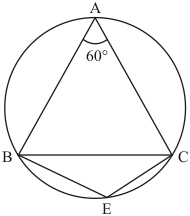It is given that,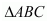is an equilateral triangleWe have to find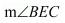Sinceis an equilateral triangle.
So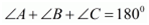And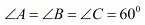…… (1)
Since, quadrilateral BACE  is a cyclic qualdrilateral

So ,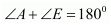(Sum of opposite angles of cyclic quadrilateral is .)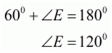Hence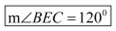Question 2 : In the given figure, ΔPQR is an isosceles triangle with PQ = PR and m ∠PQR = 35°. Find m ∠QSR and m ∠QTR.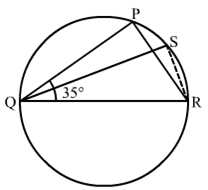Disclaimer: Figure given in the book was showing m∠PQR as m∠SQR.
It is given that ΔPQR is an isosceles triangle with PQ = PR and m∠PQR = 35°We have to find the m∠QSR and m∠QTR
Since ΔPQR is an isosceles triangle
So ∠PQR = ∠PRQ = 35°
Then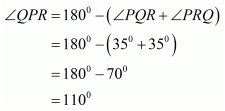Since PQTR is a cyclic quadrilateral
So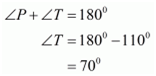In cyclic quadrilateral QSRT we have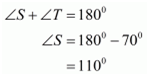Hence,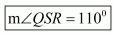and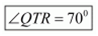Question 3 : In the given figure, O is the centre of the circle. If ∠BOD = 160°, find the values of x and y.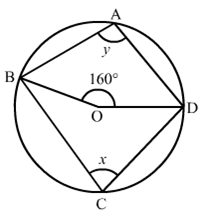It is given that O is centre of the circle and ∠BOD = 160°We have to find the values of x and y.
As we know that the angle subtended by an arc of a circle at the centre is double the angle subtended by it at any point on the remaining part of the circle.
Therefore,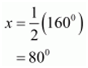So,
x + y = 180°              (Sum of opposite angles of a cyclic quadrilateral is 180°.)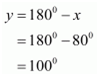Hence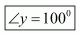and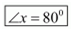Question 4 : In the given figure, ABCD is a cyclic quadrilateral. If ∠BCD = 100° and ∠ABD = 70°, find ∠ADB.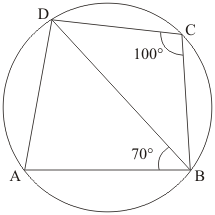It is given that ∠BCD = 100° and ∠ABD = 70°We have to find the ∠ADB
We have
∠A + ∠C = 180°                     (Opposite pair of angle of cyclic quadrilateral)
So,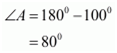Now in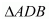is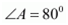and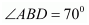Therefore,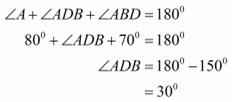Hence,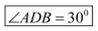Question 5 : If ABCD is a cyclic quadrilateral in which AD || BC (In the given figure). Prove that ∠B = ∠C.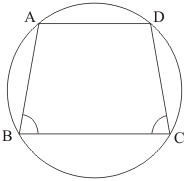It is given that, ABCD is cyclic quadrilateral in which AD || BCWe have to prove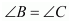Since, ABCD is a cyclic quadrilateral
So,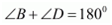and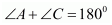..… (1)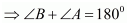and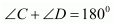(Sum of pair of consecutive interior angles is 180°) …… (2)
From equation (1) and (2) we have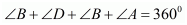…… (3)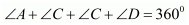…… (4)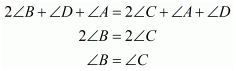Hence Proved

Question 6 : In the given figure, O is the centre of the circle. Find ∠CBD.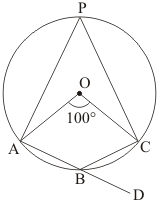It is given that,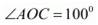We have to find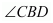Since,(Given)
So,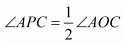(The angle subtended by an arc of a circle at the centre is double the angle subtended by it at any point on the remaining part of the circle.)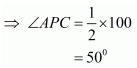Now,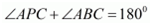(Opposite pair of angle of cyclic quadrilateral)
So,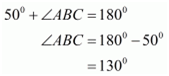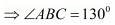…… (1)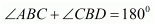(Linear pair)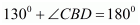(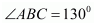)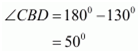Hence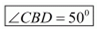Question 7 : In the given figure, AB and CD are diameters of a circle with centre O. If ∠OBD = 50°, find ∠AOC.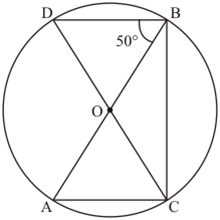It is given that, AB and CD are diameter with center O and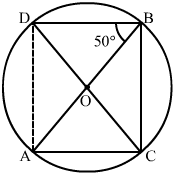We have to find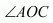Construction: Join the point A and D to form line AD
Clearly arc AD subtends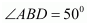at B and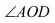at the centre.
Therefore,  ∠AOD=2∠ABD=100°∠AOD=2∠ABD=100° …… (1)
Since CD is a straight line then

∠DOA+∠AOC=180°        (Linear pair)∠DOA+∠AOC=180°        Linear pair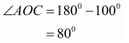Hence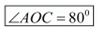Question 8 : On a semi-circle with AB as diameter, a point C is taken, so that m (∠CAB) = 30°. Find m (∠ACB) and m (∠ABC).

It is given that,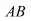as diameter,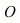is centre and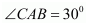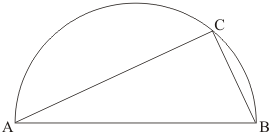We have to find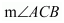and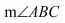Since angle in a semi-circle is a right angle therefore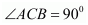In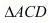we have(Given)(Angle in semi-circle is right angle)
Now in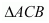we have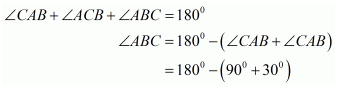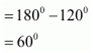Hence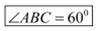and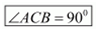Question 9 : In a cyclic quadrilateral ABCD if AB || CD and ∠B = 70°, find the remaining angles.

It is given that, ABCD is a cyclic quadrilateral such that AB || CD and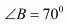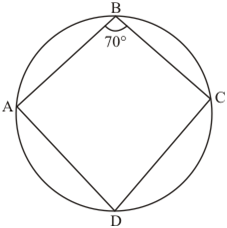Sum of pair of opposite angles of cyclic quadrilateral is 180°.(  given)
So,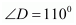Also AB || CD and BC transversal
So,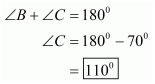Now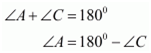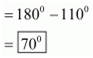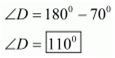Question 10 : In a cyclic quadrilateral ABCD, if m ∠A = 3 (m ∠C). Find m ∠A.

It is given that
ABCD is cyclic quadrilateral and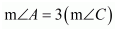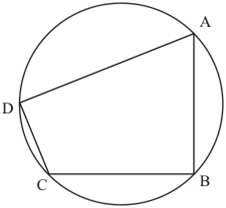We have to find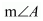Since ABCD is cyclic quadrilateral and sum of opposite pair of cyclic quadrilateral is 180°.
SoAnd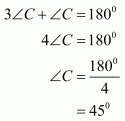Therefore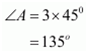Hence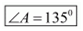Todays Deals